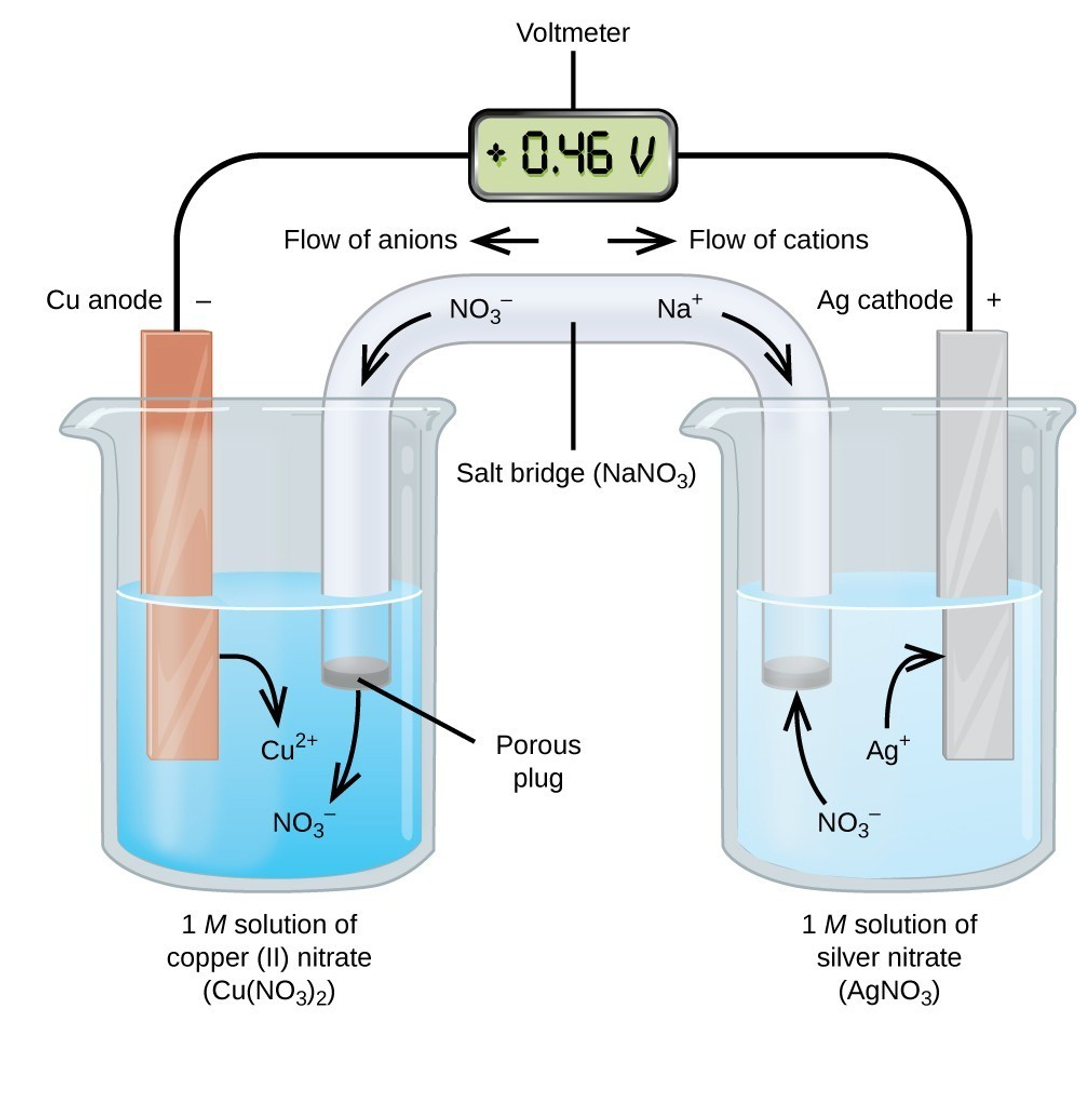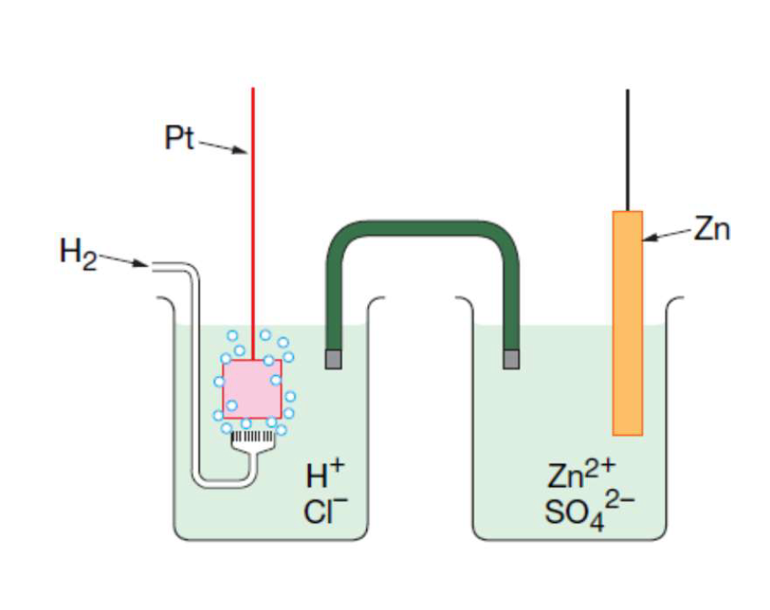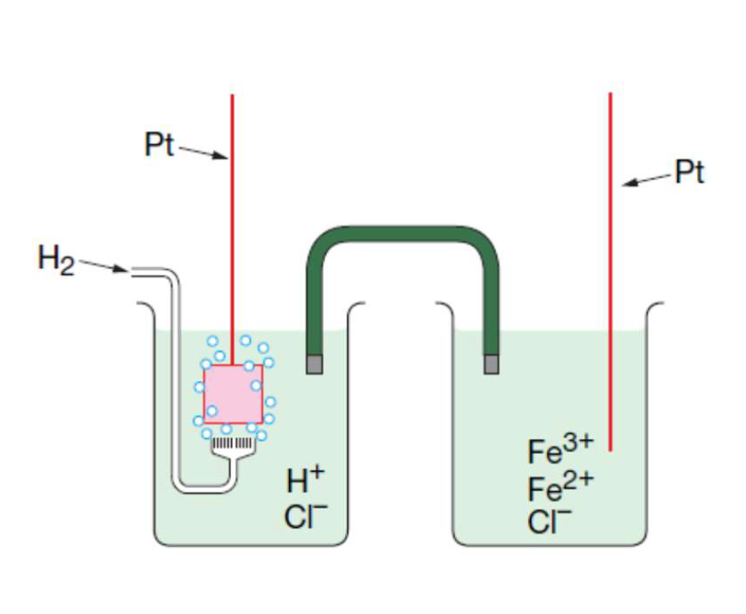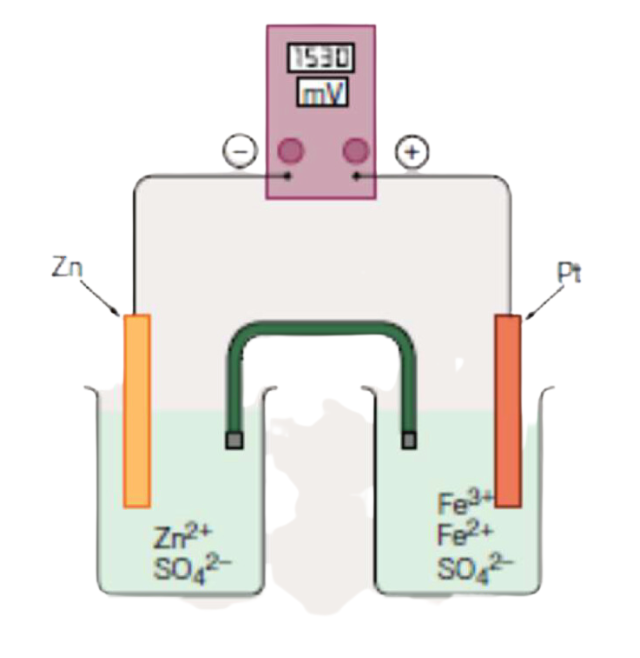# Galvanic Cells

This is part of Year 11 HSC Chemistry course under the topic of Predicting Reactions of metals

### HSC Chemistry Syllabus

• Apply the definitions of oxidation and reduction in terms of electron transfer and oxidation numbers to a range of reduction and oxidation (redox) reactions
• Conduct investigations to measure and compare the reaction potential of galvanic half-cells
• Construct relevant half-equations and balanced overall equations to represent a range of redox reactions
• Predict the reaction of metals in solutions using the table of standard reduction potentials
• Predict the spontaneity of redox reactions using the value of cell potentials (ACSCH079, ACSCH080)

### Galvanic Cells

This video discusses galvanic cells in terms of the way that they operate to produce a voltage and both oxidation and reduction reactions.

### Components of a Galvanic cell

A galvanic cell comprises several key components

• Electrodes: Electrodes are conductive materials which facilitate the oxidation and reduction reactions of a galvanic cell
• Salt Bridge: A crucial component of a galvanic cell is the salt bridge which maintains electrical neutrality through allowing ions to flow and balance charges during the reaction. It also completes the circuitIn the example of a galvanic cell above, copper and silver act as the electrodes while the salt bridge contains sodium nitrate solution.

### Displacement Reactions and Electrode Processes

When a more reactive metal is dipped into a solution of a less reactive metal's ions, a displacement reaction occurs, leading to the deposition of the less reactive metal. This process is integral to the functioning of a Galvanic cell.

For example, copper (more reactive), placed into a silver ion solution (less reactive), leads to copper oxidisation and silver reduction – observable by the development of a characteristic blue colour from the copper ions and the deposition of silver on the copper electrode.

### Oxidation and Reduction Halves

In Galvanic cells, oxidation and reduction reactions occur simultaneously while separated into two different beakers connected by a salt bridge. Oxidation occurs at the anode and reduction occurs at the cathode. .

### Calculating EMF of Galvanic Cells (Review)

The electromotive force (EMF) of a cell is the voltage developed but he redox reaction. Using the standard potential table which is provided to you on the NESA data sheet:

The copper (Cu) oxidation potential: –0.34V

The silver (Ag) reduction potential: +0.80V

Total EMF produced by this cell is +0.46V, indicating a spontaneous reaction as detailed in our video on redox reactions.

### The Role of a Salt Bridge

Using our example galvanic cell consisting of copper and silver as shown in the diagram above, we can observe the accumulation of negative charge at the anode and positive charge at the cathode.

Anode equation:

$$Cu(s) \rightarrow Cu^{2+}(aq) + 2e^–$$

The oxidation of copper leads to loss of electrons. Ultimately there is a net gain of electrons in the oxidation half-cell.

Cathode equation:

$$Ag^{+}(aq) + 2e^– \rightarrow Ag(s)$$

The reduction of silver leads to the gaining of electrons. Ultimately there is a net loss of electrons in the reduction half cell.

Because of the electrical charge imbalance which is experienced by the two half cells, respective positive and negative ions from the salt bridge enter the solution to neutralise charge.

This means that for the beaker which contains copper, Na^+ ions which carry a positive charge will enter the system to neutralise the excess negative charge, while for the beaker which contains silver,  NO_3^– ions will enter the system to neutralise the excess positive charge

### Galvanic Cell Notation

Galvanic cells have a specific shorthand notation that succinctly represents the setup of the electrochemical cell.

Understanding the symbols:

• Single Vertical Line (|): This symbol is used to separate different states of matter within the same half-cell. For example, a solid electrode from its aqueous ions or an aqueous ion from a gas.
• Double Vertical Lines (||): These lines represent the salt bridge within the cell.

For instance:

$$Cu(s) | Cu^{2+}(aq) || Ag^{+}(aq) | Ag(s)$$

The left-hand side demonstrates the anode (copper) where oxidation occurs. the single vertical line separates the solid electrode from its aqueous ions. The double vertical lines indicate the presence of a slat bridge between the two half-cells, allowing for ion exchange. The silver ions in aqueous state and the single vertical line next to it similarly indicates the separation of the silver ions from the solid silver electrode. The right-hand side indicates the cathode (silver).

### Chemical to Electrical Energy Conversion

Galvanic cells efficiently convert chemical energy into electrical energy through metal-ion reactions. Sometimes, inert electrodes like graphite or platinum are used when redox components cannot serve as electrodes themselves.

### Practice Questions and Examples (Answered in Video)

Example 1: write half-cell equations for this galvanic cell and calculate its standard potentialDirection of electron flow: Anode to Cathode

Notation: $$Pt(s) | H^+(1M) | H_2(g) || Zn(s) | Zn^{2+}(aq)$$

Standard potential:

$$H_2(g) \rightarrow 2H^+(aq) + 2e^– \text{V = 0.00}$$

$$Zn^{2+}(aq) + 2e^– \rightarrow Zn(s) \text{V = –0.76}$$

$$\text{Total EMF: } 0.00V + (–0.76V) = –0.76V$$

Example 2: Write half-cell equations for this galvanic cell and calculate its standard potentialNotation: $$Pt(s) | H^+(1M) | H_2(g) || Fe^{3+}(aq) | Fe^{2+}(aq) | Pt(s)$$

Standard potential:

$$H_2(g) \rightarrow 2H^+(aq) + 2e^– \text{V = 0.00}$$

$$Fe^{3+}(aq) + e^– \rightarrow Fe^{2+}(aq) \text{V = 0.77}$$

$$\text{Total EMF: } 0.00V + 0.77V = 0.77V$$

Example 3: Write the half-cell equations for this galvanic cell and calculate its standard potentialNotation: $$Zn(s) | Zn^{2+} || Fe^{3+}(aq) | Fe^{2+}(aq) | Pt(s)$$

Standard potential:

$$Zn(s) \rightarrow Zn^{2+}(aq) + 2e^– \text{V = 0.76}$$

$$Fe^{3+}(aq) + e^– \rightarrow Fe^{2+}(aq) \text{V = 0.77}$$

$$\text{Total EMF: } 0.76V + 0.77V = 1.53V$$

Example 4: The following reaction takes place in an electrochemical cell.

$$Cu(s) | Cu^{2+} || Fe^{3+}, Fe^{2+} | Pt(s)$$

Draw a diagram to represent this galvanic cell.

(Refer to Video)